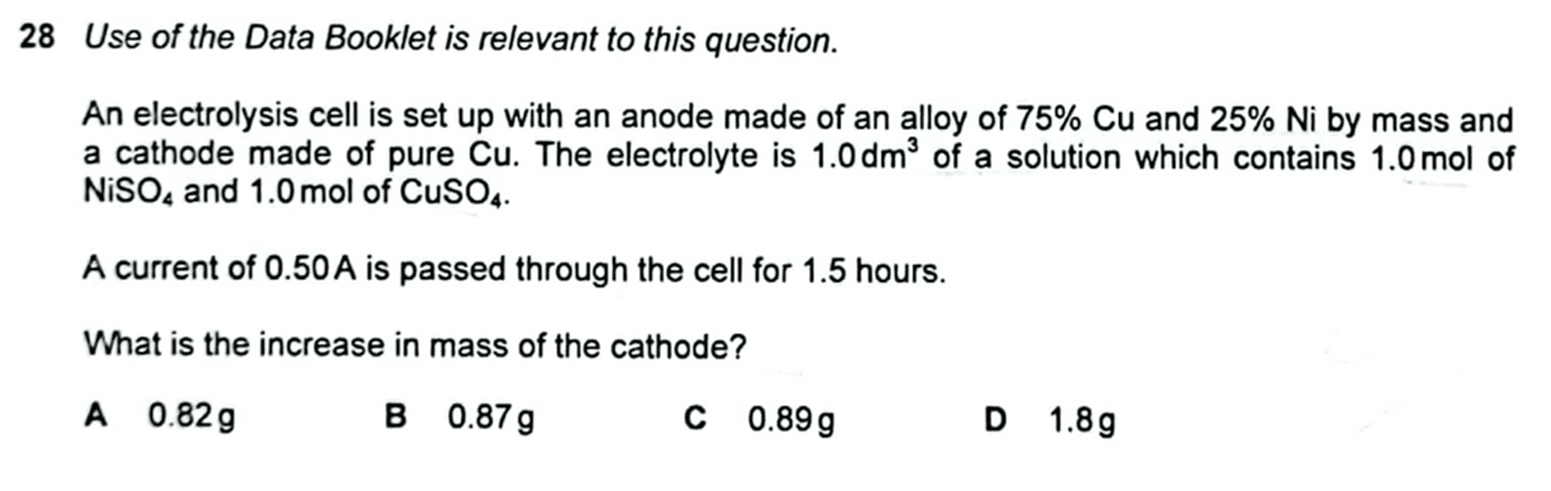# 2021 P1 Q28 - Calculate Increase in Mass of Cathode during Electrolysis

Let's take a look at 2021 A Levels H2 Chemistry Paper 1 Question 28.We are required to determine the increase in mass of cathode.

First let us calculate the number of moles of electrons involved during this electrolysis using Faraday's equations.Next we have to deduce the reaction at the cathode.

Since electrolyte is 1.0 moldm-3 of Cu2+ and Ni2+, we have to compare their standard electrode potentials and determine which is more likely reduced at the cathode.Since Cu2+ has a more positive E value, it is more likely reduced than Ni2+.

Therefore Cu2+ will be reduced at the cathode.Now we can compare mole ratio between copper and electrons to determine moles and mass of copper deposited at the cathode.Hence the answer to this question is option C.Topic: Electrochemistry, Physical Chemistry, A Level Chemistry, Singapore

Back to list of questions for 2021 A Level H2 Chemistry Paper 1.

Found this A Level Chemistry video useful?